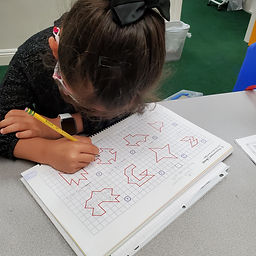## Ms. Raejean

### Target 1​

###### Lesson Type:

Review

Number Operation

:

Computation

Divide within 100.

###### 1:

Understand that dividing numbers makes numbers smaller.

###### 2:

Understand that division represents taking a quantity and determining how many equal groups of objects can be made.

###### 3:

Understand that division can be represented with repeated subtraction.

###### 4:

Understand that division equations can be written both vertically and horizontally and division can be indicated using multiple symbols (i.e., ÷, ⟌).

3rd

###### Vocabulary:

Division, Subtracting, Equal Groups

Activities:

We reviewed division. Students practiced subtracting equal groups. Ex. 20 ➗ 5 (20 - 5 = 15 - 5 = 10 - 5 = 5 - 5 = 0, 5 was subtracted 4 times) Students practiced dividing objects into equal groups. We discussed the meaning of the equation. Ex. 20 ➗ 4 means to divide the objects into groups of 4 (5 groups of 4) OR fair sharing 20 objects into 4 groups (5 in each group). We also talked about the 2 ways to write a division equation (horizontally & vertically).### Home Exploration

###### Guiding Questions:## Absent Students:

### Target 2

:

###### 1:

Relate area to arithmetic operations (multiplication and addition).

###### 2:

Recognize area as a measurement of 2D shapes.

3rd

###### Vocabulary:

Area, Square Units, Halves

Activities:

Students learned to find the area of 2D shapes. To find the area, students counted the number of squares that fit on the shape, including the halves.### Home Exploration

###### Guiding Questions:### Target 3

:

###### Vocabulary:

Activities:### Home Exploration